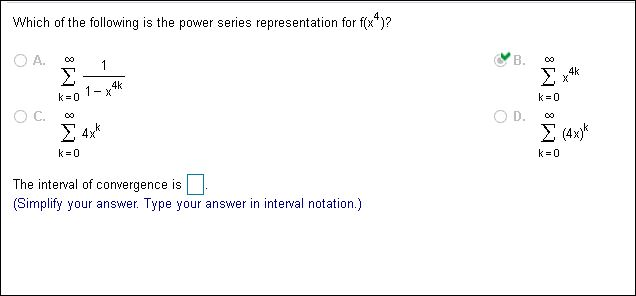# Which of the following is the power series representation for f(x)? O A. B. 1 4k X Σ 4k k 0 1-x k 0 D. Σ4 .k Σ 4x k 0 k 0 The interval of convergence is (Simplify your answer. Type your answer in interval notation.) 8 8 8 8

Questionhelp_outlineImage TranscriptioncloseWhich of the following is the power series representation for f(x)? O A. B. 1 4k X Σ 4k k 0 1-x k 0 D. Σ4 .k Σ 4x k 0 k 0 The interval of convergence is (Simplify your answer. Type your answer in interval notation.) 8 8 8 8 fullscreen

### Want to see this answer and more?

Step-by-step answers are written by subject experts who are available 24/7. Questions are typically answered in as fast as 30 minutes.*